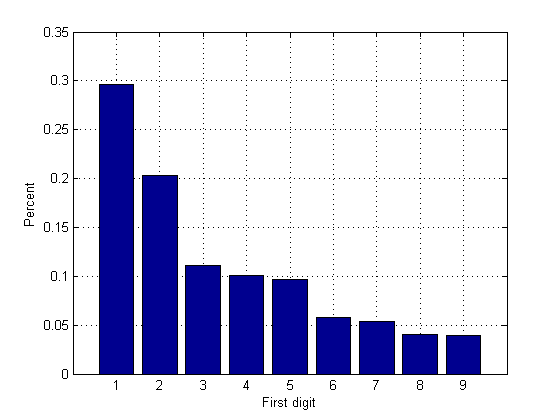## Loren on the Art of MATLABTurn ideas into MATLAB

Note

Loren on the Art of MATLAB has been archived and will not be updated.

# Benford’s Law – What are the odds that the first digit is a ‘1’?

I'd like to introduce this week's guest blogger Sam Mirsky. Sam is an Application Engineer here at MathWorks who focuses on real-time testing applications using Simulink. However, in this post he will talk about a non-intuitive characteristic of large data sets, and test the idea with a data set which ships with MATLAB.

In a large set of data, it seems that the probability of individual numbers starting with 1 would be the same as any other digit. However, this is not true. There is a much higher probability that the first digit is a 1.

Since the first significant digit is not zero, the intuitive probability of a number starting with 1 (or any other digit) would be 1/9 = 11%. According to Wikipedia: "The first digit is 1 about 30% of the time, and larger digits occur as the leading digit with lower and lower frequency, to the point where 9 as a first digit occurs less than 5% of the time."

### Contents

Let us test this with a data set which ships with MATLAB: quake.mat. This is a data set with accelerometer data from an earthquake in California.

load quake

### Find digit statistics

stat(1:9) = 0;
for i = 1:length(v)
string = sprintf('%0.5e', abs(v(i)));
firstDigit = str2double(string(1));
switch firstDigit
case 1
stat(1) = stat(1) +1;
case 2
stat(2) = stat(2) +1;
case 3
stat(3) = stat(3) +1;
case 4
stat(4) = stat(4) +1;
case 5
stat(5) = stat(5) +1;
case 6
stat(6) = stat(6) +1;
case 7
stat(7) = stat(7) +1;
case 8
stat(8) = stat(8) +1;
case 9
stat(9) = stat(9) +1;
end
end

### Plot results

statPercent = stat / sum(v ~= 0);  %only use non-zero numbers for stats
bar(statPercent);
grid on;
xlabel('First digit');
ylabel('Percent');### How this is used

This is one test that is done to test if a data set is real or fabricated. For example, if you collect all the numbers on a federal income tax return, it should also obey Benford's Law.

### How would you use MATLAB to calculate these statistics?

As is typical with MATLAB, there are many ways to derive the same answer:

• What MATLAB commands would you use to analyze the first digit of numbers in a data set?
• Does Benford's Law apply to a data set you have (or not)? Show us your results here.

Published with MATLAB® 7.14